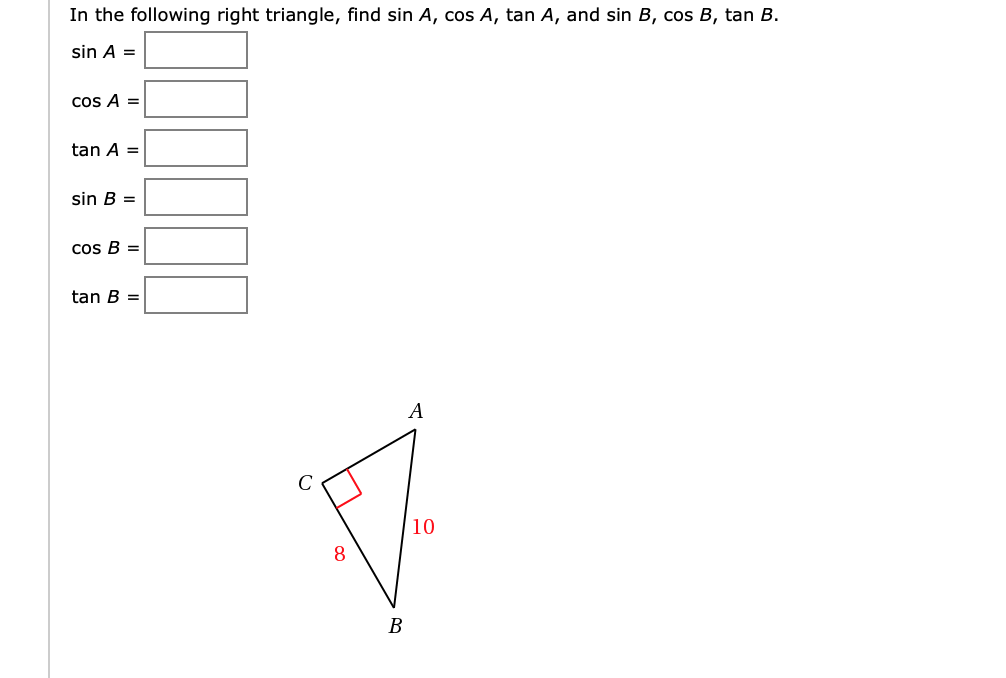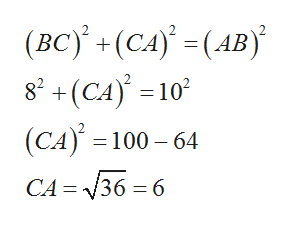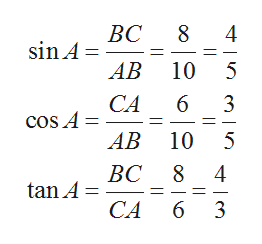In the following right triangle, find sin A, cos A, tan A, and sin B, cos B, tan B.sin A =COS Atan Asin BCOs Btan B10B

Questionhelp_outlineImage TranscriptioncloseIn the following right triangle, find sin A, cos A, tan A, and sin B, cos B, tan B. sin A = COS A tan A sin B COs B tan B 10 B fullscreen
Step 1

Consider the given right triangle ABC , where angle C is right angle.

In order to find the trigonometric ratios from right triangle, first compute the height of the triangle using Pythagorean theorem as follows.help_outlineImage Transcriptionclose(BCy(CA) (AB 8+(CA10 (CA) 100 64 CA 36 6 fullscreen
Step 2

The given trigonometric ratios from right triangle referenc...help_outlineImage Transcriptionclose8 4 ВС sin A AB 10 5 CA 6 3 coS A АВ 10 ВС 8 5 4 tan A CA 6 3 fullscreen

Want to see the full answer?

See Solution

Want to see this answer and more?

Our solutions are written by experts, many with advanced degrees, and available 24/7

See Solution
Tagged in

Trigonometric Ratios